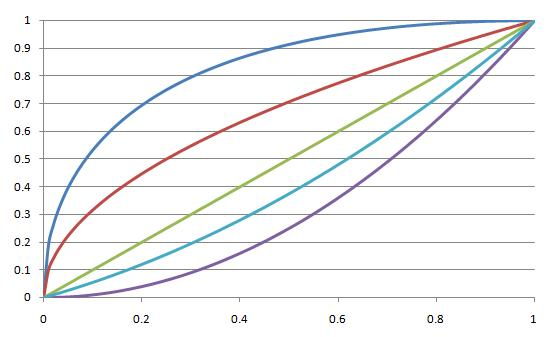#### You may also like### How Many Solutions?

Find all the solutions to the this equation.### Quartics

Investigate the graphs of y = [1 + (x - t)^2][1 + (x + t^)2] as the parameter t varies.### Power Up

Show without recourse to any calculating aid that 7^{1/2} + 7^{1/3} + 7^{1/4} < 7 and 4^{1/2} + 4^{1/3} + 4^{1/4} > 4 . Sketch the graph of f(x) = x^{1/2} + x^{1/3} + x^{1/4} -x

# Curve Match

##### Age 16 to 18Challenge Level

The following five functions have been plotted accurately on the chart below
$$y=\sqrt{x}\quad\quad y=x^2\quad\quad y=x\quad\quad y=(x^2+x)/2\quad\quad y=2\sqrt{x}-x$$Which is the $x$-axis and which is the $y$-axis? Which curves correspond to which functions?

Imagine that you wish to plot a route between $(0, 0)$ and $(1, 1)$. Can you find a function which does this without intersecting any of the existing curves, except at the endpoints?

What other curves can you plot which only intersect the existing curves at the end points?